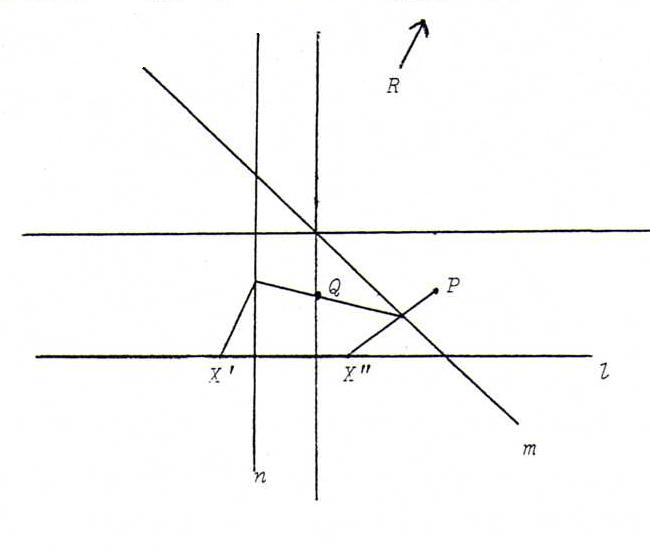### COURSE OF PROJECTIVE GEOMETRY

§ 5: answers

O17
i) λ(a2b3-a3b2,a3b1-a1b3,a1b2-a2b1), short: λab. Indeed, ab is normal of the plane subtended by a and b.
ii) lm. Indeed, lm is direction vector of the intersecting line of two planes with normals through l and m, respectively.

O18
i) det(a,b,c)=0. Indeed, this is the condition that c belongs to the plane subtended by a and b.
ii) det(l,m,n)=0. Indeed, three planes through the origin go through one line if their normals lie in one plane.

O19 If det(aa',bb',cc')=0 then det((ab)⊗(a'⊗b'),(ac)⊗(a'⊗c'),(bc)⊗(b'⊗c'))=0.

O20 If det(a1,a2,a3) = det(b1,b2,b3) = 0, then det((a1b2)⊗(a2b1),(a1b3)⊗(a3b1), (a2b3)⊗(a3b2)) = 0.

O21 Start from λ(a,b,c) on l, this is λ(a,-2,1) or λ(1,0,0). The image point is λ(13-3a,16-4a,2a-8). This is the point λ(a,-2,1) if 3a-13 = a(8-2a). We get a =(5+√129)/4.